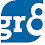# Simple Method for Calculating Shortcut for Square - Part 2

We've already seen how to find the squares of numbers which are one more than those whose squares we already know (eg 25, 30, 35 etc).Read that post Here. Similarly, we can find the squares of numbers which are one less than the numbers whose squares are known already to us. For Example,
292 = 30 - 30th odd number = 900 - 59 = 841
392 = 40 - 40th odd number = 1600 - 79 = 1521
342 = 1225 - 69 = 1156
542 = 3025 - 109 = 2916
742 = 5625 - 149 = 5476
942 = 9025 - 189 = 8836
2142 = 46225 - 429 = 45796

So, we have seen how to get the squares of numbers which are one more or one less than the numbers whose squares we already know (i.e., 25, 30, 35, 40, etc)

Now, we shall see how to get the squares of numbers which are 2 more or less than the numbers whose squares we already know.

272 = 262 +  27th odd number = 252 + 26th odd number + 27th Odd number.

The sum of the 26th odd number and 27th odd number is the same as 4 times 26

So,

272  = 252 + 4x26 = 625 + 104 = 729
57 = 3025 + 224 (4 times 56) = 3249
77 = 5625 + 304 (4 times 76) = 5929
97 = 9025 + 384 (4 times 96) = 9409

Similarly,  we can find out the squares of numbers which are 2 less than the numbers whose squares we know

28= ( 30 - 4 times 29) = 900 - 116 = 784
53 = (55- 4 times 54) = 3025 - 216 = 2809
93 = 9025 - 376 = 8649
2432 = 60025 - 976 = 59049

Note : You should do all these calculations MENTALLY. I mean without using pen or pencil. So practice well to do these problems orally.

Liked this post ?? Read More Here >>

1.square of 53 is 2809 and not 2089...kindly corect it..i think its printing mistake..

1.Yep its a typing mistake friend. Corrected it now :)
Sorry for the inconvenience..

2.A GENERALIZED FORMULA CAN BE DRAWEN
for no ending 6 and 1
(n-1)^2+(2n-1)
for no ending 4 and 9
(n+1)^2-(2n+1)

3.confused with 74²
..actually to find odd number we use (2n-1), therfore 74²=75²-74th odd number
5625-(2*74-1)=5625-(148-1)
therefore answer should be 5578 which is wrong but u have taken 149...hw is that plz explain

4.Sorry i got that.. i should have taken 75th odd number but i made mistake by taking 74th :(

Thanks :)